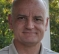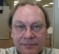## find out frequency of complex sinusoidal without FFT or DFTStarted by 5 years ago11 replieslatest reply 5 years ago438 views

I have a complex sinusoidal signal (discrete samples as in-phase/real and quadrature phase/imag componenet) in DSP, whose frequency (single frequency) is in few 10's or 100's of Hz. My sampling rate is few 100s of kHz. Let's say Number of samples available for analysis are 100.

What are the algorithm to estimate the exact or approximate (with known deviation) frequency without going for FFT or DFT?

I am trying to implement this in 'C' and I have very little room for complex algorithm (v MIPS to approximate the frequency of the signal.

What will be the performance of the algorithm chosen in presence of noise?

[ - ]Fairly simple answer for waveforms with relatively clean tones.

Simply demodulate successive samples against each other:

y = x(n)*conj(x(n-1));

If you normalize the abs(y) then the phase change per sample is approximately eq to imag(y/abs(y));  (Good only for low frequencies relative to sampling rate).

Use atan2(imag(y),real(y)) for the accurate result.

Now with the know sampling rate, you can figure out the freq = fs*phase(y)/2*pi.  (Corrected as per mukul, thank you)

Averaging of the phase(y) will give more accuracy, and resistance to noise.

If you are looking for frequencies within a certain range, then run a BPF on x(n) before the demodulation.

---------

If you are looking for some specific tones, whose frequencies are know a priori, then run Goertzel detectors for the frequencies you care about.

I mention this because you reference 50 Hz and 100 Hz, which I assume are international power line components.

Goertzel is nice on complex signals, because it is a simple complex multiply with one feedback.  However, you will need to do integrate and dump periodically, or the internal variables in the Goertzel will grow without bound.

The integrate and dump time will define the discrimination between tones.  Also, if the integrate and dump time is too long, and you need results more often, then run multiple Goertzels at the same frequency with staggered start times, so that one of them is expiring at a more frequent interval.

[ - ]Hi @dgshaw6,

Thanks for the detailed suggestion. It worked but I am still searching for something more simpler where I don't need to use even atan2 for finding out phase of each sample. Even atan2 is quite costly considering the computational capability I have and a very simplified case of frequency estimation I am looking into.

Just one thing you might like to update your answer as freq will not be fs*2*pi*phase(y) but fs*phase(y)/(2*pi). As the sample time difference between two consecutive sample is 1/fs and phase difference within those samples difference = 2*pi*f(1/fs)

[ - ]Thanks for the correction to an obvious error in my first response. I have attempted to make the correction above.

I took a trip down a deep rabbit hole as I was looking at several of the other responses to you question.  In one of the comments posted to the original article from Rick. (Comment from dszabo April 27 2017):

In the comment, dszabo references a brilliant technique of Rick's, from a chapter of his book made available through the embedded website.

https://www.embedded.com/design/configurable-systems/4212086/DSP-Tricks--Frequency-demodulation-algorithms-

In Figure 16-31(b) there is a technique outlined for delta(phase) that has no transcendental functions in it.  This will save you either the atan2, or the arccos required by some of the other techniques discussed in other articles referenced in other answers given here.

[ - ]Hello mukel.

Perhaps the following blog will be of some help to you. (Who knows?)

[ - ]Or my generalized followup articles:

They will allow you to include more points to affect some noise reduction.

[ - ]Hi Rick,

This exactly what @dgshaw6 suggested in a formal article.

And yes it helped. Thanks a lot.

By the way my name is Mukul and not Mukel

[ - ]Hi Mukul. Sorry for my clumsy typing.

[ - ]If you have enough computational power to find an autocorrelation lag, then use Pisarenko Harmonic Decomposition (or any of several other eigenspace algorithms such as Prony's algorithm or Matrix Pencil).

For a single sinusoid in uncorrelated noise it is computationally inexpensive - no need to root high order polynomials, and certainly no need to find peaks of FFTs or ARMA models.

The reason that PHD is better than FM demodulation because it is a "super-resolution" method - the accuracy mostly depends on the inverse square of the SNR rather than being precision-limited by the uncertainty theorem.

If you can't afford autocorrelation, then try a poor-man's PHD. Just divide each complex sample by the previous complex sample to get an estimate of e^iw = cos(w) + i sin(w). Inverse cos or a division and inverse tan retrieves w. Averaging a few will improve accuracy, although this averaging after the inverse trig function isn't the right thing to do.

Y(J)S

[ - ]First, this depends on what is meant by "complex" sinusoid? I'm guessing the intended meaning is that the signal contains sine waves of multiple frequencies. If so, that (to me) complicates the matter, since the typical way to measure a single sine wave's frequency is to determine the number of samples between "zero" crossings, true only if there is no (significant) DC offset. There may be other ways to estimate the frequency if you know the range of frequencies a priori. Even for a single frequency, the accuracy is dependent on the number of samples between the zero crossings. The best way to address accuracy, is to average the frequency estimate of (a type of low-pass filter) successive samples. With enough complications, this may lead to tossing in the towel and just using an FFT, which is computationally efficient, but it too may only be a rough estimate.

As always, the most critical part of any implementation, is making sure the problem statement is 100% correct and unambiguous. Then weigh the benefits of available alternate methods by analysis.

[ - ]Hi @artmez,

Thanks for the quick answer, I have edited my question but I am clarifying again here.

It's a single frequency tone of lets say 50 or 100 Hz.

By complex Sinusoidal I meant that I don't have real samples but complex I and Q component in my baseband (which show spectra only on one side of DC).

I know that same zero crossing could be used for one of the inphase or quadrature phase component of complex sinusoidal as of any real samples of sinusoidal but here in my case frequency component is near to DC comparing to sampling rate and number of samples and I wouldn't get many zero crossing. Further in presence of noise counting zero crossing will not give good performance. Even if I use FFT (which I can't due to MIPS complexity) I don't get exact resolution because of less number of analysis samples available. Zero padding for better resolution further demands for higher order FFT.

Now regarding the apriori knowledge I have is:

1. Signal is in Hz and sampling rate is in kHz

2. Samples are of a single tone complex sinusoidal

3. Full blown FFT can't be used

[ - ]